# HC Verma Solutions Class 12 Chapter 9 Capacitor

HC Verma Solutions Class 12 Chapter 9 Capacitor is available here which students can make use of to solve different types of problems and exercises in the most comprehending manner. The solutions that we are proving here have been thoughtfully written and are designed to offer seamless preparation.

Students will come across mathematical equation such as C= Q/ V where C is the capacitance, Q is the stored electric charge, V is the voltage between the plates of a capacitor. They will also have to be familiar with formulas that are used in different instances. Some of them are – To measure the capacitance of capacitors in series: 1/Ctotal = 1/C1 + 1/C2 + 1/C3 +…1/Cn. To measure the capacitance of capacitors in parallel: Ctotal=C1+C2….Cn. The energy of a capacitor is given by EC = C × VC2 / 2.

The HC Verma Solutions will be an invaluable aid to students who are especially gearing up to appear for competitive exams like JEE. Students can use these solutions as a reference tool to understand the concepts in depth and learn the right method to solve questions given in the HC Verma book. In essence, students will be well prepared to tackle any type of physics question that may be asked in the exam.

## Fundamental Topics Related To Capacitor

The chapter includes exercises and questions based on topics like;

• Capacitance
• Force Between the Plates of a Capacitor
• Energy Stored in a Capacitor
• Electric Field due to Charge q
• Corona Discharge
• High-voltage Generator
• Dielectrics

## Class 12 Important Questions In Chapter 9

1. State some of the factors that the capacitance of a capacitor does not depend on?

2. Two metal plates are dipped in an oil tank. Both the plates have charges Q – Q and are facing each other at some separation. What will happen to the electric field if the oil is pumped out?

3. When a dielectric slab is inserted between the plates of an isolated capacitor what will happen to the force between the plates?

(a) It will become zero (b) increase (c)  decrease (d) remain unchanged

4. A capacitor has parallel plates which are of unequal area. The larger plate is connected to the positive terminal of the battery and the smaller plate to the negative terminal. If the charges present are given as Q, and Q that appear on the positive and negative plates respectively. Choose the right answer.

(a) Q = Q (b) Q > Q (c) Q < Q (d) Cannot determine the relation as the information is not sufficient

5. If we increase the separation between the plates of a charged parallel plate capacitor, what quantities will change?

(a) potential difference across the capacitor (b) energy density between the plates (c) energy of the capacitor (d) charge on the capacitor

## HC Verma Solutions Vol 2 Capacitor Chapter 9

Question 1: When 1.0 × 1012 electrons are transferred from one conductor to another, a potential difference of 10V appears between the conductors. Calculate the capacitance of the two-conductor system.

Solution:

The capacitance of a parallel plate Capacitor : C = Q/V …(1)

Here, C = capacitance of the parallel plate capacitor

Where Q is the charge on the capacitor and V is the Voltage or potential difference.

Also, we know, Q = ne = (1 x 1012) x (1.6 x 10-19) = 1.6 x 10-7 C

Therefore,

(1) => C = [1.6 x 10-7]/10 = 1.6 x 10-8 F

Question 2: The plates of a parallel-plate capacitor are made of circular discs of radii 5.0 cm each. If the separation between the plates is 1.0 mm, what is the capacitance?

Solution:

Since capacitor plates are made of circular discs, so A = area of the circle = πr2.

Here r = 5 x 10-2

=> A = π(5 x 10-2)2

We know, Capacitance of the capacitor = C = ЄoA/d

Where, A = area of the plate

εₒ = Permittivity of free space = 8.85 x 10-12 F/m

d = Separation between the plates

Now, C = [8.85 x 10-12x3.14x(5 x 10-2)2]/[1.0×10-3]

= 6.95 x 10-5 μF

Remember:

Parallel plate capacitor: When two conducting plates are connected in parallel and separated by some distance then parallel plate capacitor will be formed.

Question 3: Suppose, one wishes to construct a 1.0 farad capacitor using circular discs. If the separation between the discs be kept at 1.0 mm, what would be the radius of the discs?

Solution:

Here, C = 1 F and d = 1 mm

We know, Capacitance of the capacitor = C = ЄoA/d

Where, A = area of the plate

εₒ = Permittivity of free space = 8.85 x 10-12 F/m

d = Separation between the plates

Since capacitor plates are circular discs, so A = πr2

Now, C = Єo πr2 /d

Or r2 = Cd/Єo π

= [1 x (1×10-3)]/[3.14 x 8.85 x 10-12]

= 36 x 106

Or r = 6000 m = 6 Km

Question 4: A parallel-plate capacitor having plate area 25 cm2 and separation 1.00 mm is connected to a battery of 6.0 V. Calculate the charge flown through the battery. How much work has been done by the battery during the process?

Solution:

Here Area, A = 25 cm2 =25×10-4 m2

Voltage = V = 6V and d = 1mm = 1 × 10-3

When a capacitor is connected to a capacitor, the charge is Q = CV …(1)

Also, We know, Capacitance of the capacitor = C = ЄoA/d

Where, A = area of the plate

εₒ = Permittivity of free space = 8.85 x 10-12 F/m

d = Separation between the plates

=> C = [8.85×10-12x25x10-4]/[1×10-3] = 2.21 x 10-11 F

When the capacitor is connected to a battery fo voltage 6V, then flow of Q (Charge) from the battery is same as the charge that the given capacitor have.

(1)=> Q = 2.21 x 10-11 x 6 = 1.33 x 10-10 C

Work is done by the battery:

W = magnitude of the charge transferred x voltage of the battery

=> W = QV = 1.33 x 10-10 x 6 = 8.0 x 10-10 J

Question 5: A parallel-plate capacitor has plate area 25.0 cm2 and separation of 2.00 mm between the plates. The capacitor is connected to a battery of 12.0V.

a) Find the charge on the capacitor.

b) The plate separation is decreased to 1.00 mm. Find the extra charge given by the battery to the positive plate.

Solution:

a) Voltage = V = 12V ; d = 2 mm

Area = A = 25 cm2 = 25 x 10-4 m2

We know, Charge of the capacitor = Q = CV

Also, We know, Capacitance of the capacitor = C = ЄoA/d

Where, A = area of the plate

εₒ = Permittivity of free space = 8.85 x 10-12 F/m

d = Separation between the plates

C = [8.85×10-12x25x10-4]/[2×10-3] = 11.06 x 10-12 F

Now, charge on the capacitor = Q = CV = 11.06 x 10-12 x 12 = 1.33 x 10-10 C

(b)

C = [8.85×10-12x25x10-4]/[1×10-3] = 22.12 x 10-12 F

Now, charge on the capacitor = Q’ = CV = 22.12 x 10-12 x 12 = 2.652 x 10-10 C

Therefore, Extra charge = Q’ – Q = 2.652 x 10-10 – 1.33 x 10-10 = 1.33 x 10-10 C

Question 6: Find the charges on the three capacitors connected to a battery as shown in figure. Take C1 = 2.0 μF, C2 = 4.0 μF, C3 = 6.0 μF and V = 12 volts.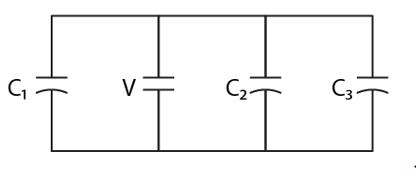Solution:

All three capacitors are connected in parallel. So, equivalent capacitance is:

Ceqv = C1 + C2 + C3 = (2+4+6) = 12 μF = 12 x 10-6 F

Let “Q” be the Charge of a capacitor, then Q = Ceqv V

Find charge on each capacitor:

Charge on capacitor C1:

Q1 = C1 x V = 2 x 12 = 24 C

Charge on capacitor C2:

Q2 = C2 x V = 4 x 12 = 48 C

Charge on capacitor C3:

Q3 = C3 x V = 6 x 12 = 72 C

Question 7: Three capacitors having capacitances 20 μF, 30 μF and 40 μF are connected in series with a 12 V battery. Find the charge on each of the capacitors. How much work has been done by the battery in charging the capacitors?

Solution:

All three capacitors are connected in series. So, equivalent capacitance is:

1/Ceqv = 1/C1 + 1/C2 + 1/C3

= C1C2C3/[C1C2 + C2C3 + C3C1]

= [20x30x40]/(20×30+30×40+40×20]

=> Ceqv = 9. 23 μF

Find charge on each capacitor:

Let “Q” be the Charge of a capacitor, then Q = Ceqv V

Q = 9.23 x 12 = 110.76 μF

Work done by the battery:

W = QV = 110.76 x 12 x 10-6 = 1.33 x 10-3 J

Question 8: Find the charge appearing on each of the three capacitors shown in the figure.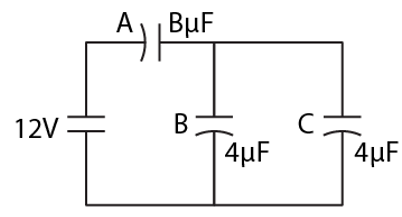Solution:

Capacitors B and C are in parallel.

So, Ceqv = CB + CC = 4 + 4 = 8 μF

Now, Ceqv and CA is series, so

1/C = 1/Ceqv + 1/CA

= 1/8 + 1/4

= 1/4

or C = 4 μF

System of A, B and C has the same capacitor values. So, exhibit the same potential difference between them.

The charge of the capacitor = Q = CV

Charge flows through A = QA = 8×6= 48μC

Charge flows through B = QB = 4×6 =24μC

Charge flows through C = QC = 4×6 = 24μC

Question 9: Take C1 = 4.0 μF and C2 = 6.0 μF in figure. Calculate the equivalent capacitance of the combination between the points indicated.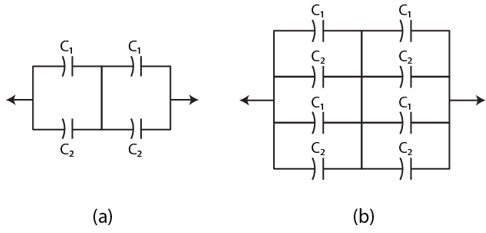Solution:

In figure, (a)

C1 and C2 are in parallel.

=> 1/Ceq = 1/(C1 + C2) + 1/(C1 + C2)

= 2/(C1 + C2)

= 2/(4+6) = 2/10 =1/5

Ceq = 5 μF

Form figure (b):

1/Ca = 1/(C1 + C2) + 1/(C1 + C2)

= 1/5

Ca = 5 μF

Also, Cb = 5 μF

Now, Ceq = Ca + Cb = 10 μF

Question 10: Find the charge supplied by the battery in the arrangement shown in the figure.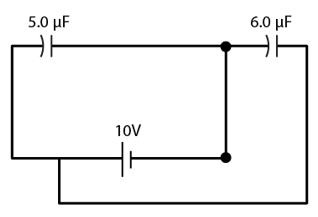Solution:

Here, C1 = 5 μF and C2 = 6 μF and V = 10 V

The equivalent circuit for the given is: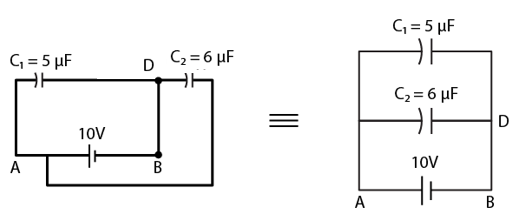Here, C1 = 5 μF and C2 are parallel, so

Ceq = C1 + C2 = 5 + 6 = 11 μF

So, Charge supplied by battery = Q = CV = 11 x 10 = 110 μC

Question 11: The outer cylinders of two cylindrical capacitors of capacitance 2.2 μf each are kept in contact, and the inner cylinders are connected through a wire. A battery of emf 10V is connected as shown in the figure. Find the total charge supplied by the battery to the inner cylinders.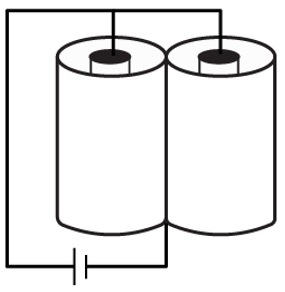Solution:

Inner cylinders are connected through a wire and Outer cylinders kept in contact. The equivalent circuit for the given case is: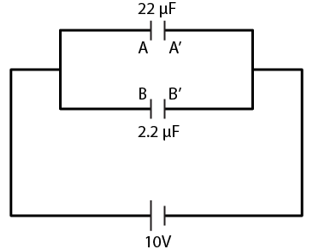Consider, inner cylinders as A and B, and outer cylinders as A’ and B’.

Now, Magnitude of the charge on each capacitor = Q = CV = 2.2 x 10 = 22 μC

Inner cylinders of the capacitor are connected to the positive terminal of the battery. The charge on both the plates is same, i.e. +22μC

Net charge on the inner cylinders is = 22μC + 22μC = +44μC

Question 12: Two conducting spheres of radii R1 and R2 are kept widely separated from each other. What is their individual capacitance? If the spheres are connected by a metal wire, what will be the capacitance of the combination? Think in terms of series-parallel connections.

Solution:

Capacitance of the charged sphere can be found by imagining a concentric sphere of infinite radius consisting of −Q charge.

The capacitance of isolated charge sphere: C = 4πЄoR

Capacitances of spheres of radii R1 and R2 are C1 and C2, respectively.

The capacitance of isolated charge sphere 1 = C1 = 4πЄoR1

The capacitance of isolated charge sphere 2 = C2 = 4πЄoR2

Since both the spheres are connected by a metal wire. So, the charge will flow one sphere to another upto their potential becomes the same when they are connected in parallel.

Ceq = C1 + C2

= 4πЄo (R1 + R2)

Question 13: Each of the capacitors shown in the figure has a capacitance of 2 μF. Find the equivalent capacitance of the assembly between the points A and B. Suppose, a battery of emf 60 volts is connected between A and B. Find the potential difference appearing on the individual capacitors.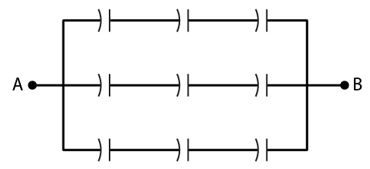Solution:

The equivalent capacitance of the first row: 1/c = 1/2 + 1/2 + 1/2 = 3/2

=> C = 2/3 μF

Similarly, capacitance of another rows is (2/3) μF

All the three rows are arranged in parallel. So, Voltage or potential difference across each row is the same and is equal to 60V.

Voltage across each capacitor = 20V

Question 14: It is required to construct a 10 μF capacitor which can be connected across a 200V battery. Capacitors of capacitance 10 μF are available, but they can withstand the only 50V. Design a combination which can yield the desired result.

Solution:

Let’s assume some X capacitors are placed in series.

So, 50x = 200

=> x = 4 C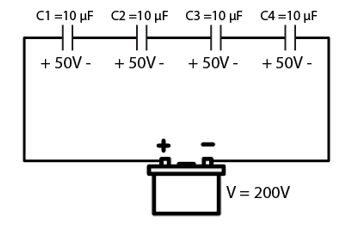Construct 4 capacitors in a series to get the potential difference of 200V.

The capacitance of the parallel combination of four 10μF capacitors:

1/c = 1/C1 + 1/C2 + 1/C3 + 1/C4 = 4/10

C = 2.5μF

To get capacitor value 10 μF instead of only 2.5μF, add some more columns. Let us take Y as columns, so

2.5 Y = 10

=> Y = 4 C

Add 4 columns as the same row, as shown below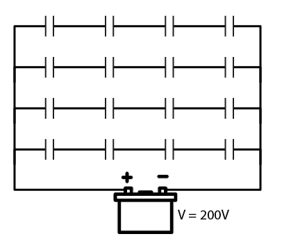Each capacitor value is 10 μF with voltage rating 50V.

Question 15: Take the potential of the point B in figure to be zero.

a) Find the potentials at the points C and D.

b) If a capacitor is connected between C and D, what charge will appear on this capacitor?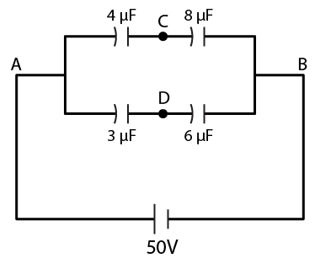Solution:

Capacitors 4μF and 8μF are in series.

C1 = 8/3 μF

Capacitors 3μF and 6μF are in series

C2 = 2 μF

C1 and C2 are in parallel combination. The voltage across each row is the same i.e. 50V.

we know, Q = CV

Now,

The Charge on the branch ACB with capacitance 8/3 μF:

Q = (8/3) x 50 = 400/3 μC

The Charge on the branch ADB with capacitance 2 μF:

Q = (2) x 50 = 100 μC

The potential at point D is

VD = q/C1 = 100/6 = 50/3 V

In same way, potential at point C is VC = 50/3 V

Question 16: Find the equivalent capacitances of the system shown in figure between the points a and b.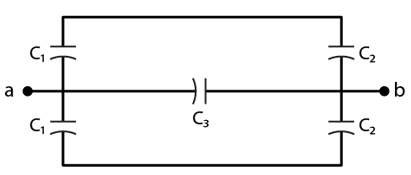Solution:

From figure, C1 and C2 are in series. So,

Equivalent capacitance = Ca = 1/C1 + 1/C2

Ca = C1C2/(C1+C2)

Similarly, Cb = C1C2/(C1+C2)

The capacitance Ca, Cb and C3 are connected in parallel combination across each other, so equalent capacitance between a and b is Ceq

Ceq = Ca + C3 + Cb

= C1C2/(C1+C2) + C3 + C1C2/(C1+C2)

= 2C1C2/(C1+C2) + C3

Question 17: A capacitor is mad of a flat plate of area A and the second plate having a stair-like structure as shown in the figure. The width of each stair is a, and the height is b. Find the capacitance of the assembly.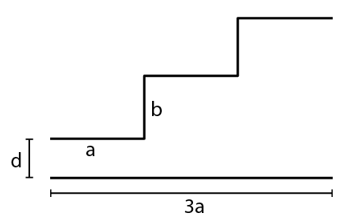Solution:

Arrange all the capacitors in parallel, the equivalent figure is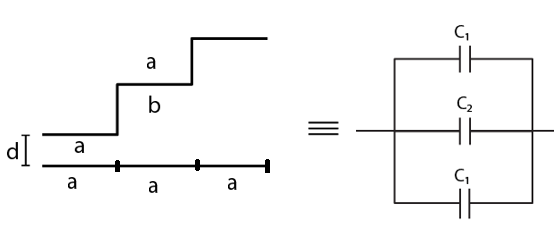Let “A” be the total area of the flat plate.

The width of each stair is the same.

Therefore, the area of each stair facing the flat plate is the same, i.e. A/3.

Here, the three capacitors are connected in parallel.

For capacitor C1: Area of the plates = A/3 and the separation between the plates is d.

For capacitor C2: Area of the plates = A/3 and the separation between the plates is (d + b).

For capacitor C3: Area of the plates= A/3 and the separation between the plates is (d + 2b).

Capacitance among all the capacitors: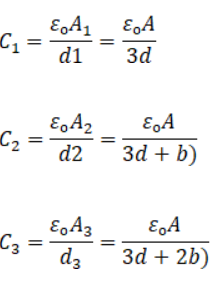Capacitors are in parallel, so capacitance of the assembly of the capacitors :

Ceq = C1 + C2 + C3

Substituting all the values. We get

Ceq = [3d2 + 6bd + 2b2]/[d(d+b)(d+2b)]

Question 18: A cylindrical capacitor is constructed using two coaxial cylinders of the same length 10 cm and of radii 2 mm and 4 mm.

a) Calculate the capacitance.

b) Another capacitor of the same length is constructed with cylinders of radii 4 mm and 8 mm. Calculate the capacitance.

Solution: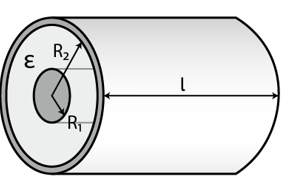(a)

We know, capacitance of the cylindrical capacitor is

C = 2πЄol/ln(R2/R1)

l= length of the cylinder

R1 = radius of inner cylinder

R2 = radius of outer cylinder

Єo = permittivity of the free space = 8.85×10-12 Fm-1

Given: R1 = 2mm ; R2 = 4mm ; l = 10cm

On putting values, we get

C = [2×3.14×8.85×10-12]/ ln(4/2) = 8 pF

(b) If we compare the radii in both the parts, we get same ratio

R2/R1 = 4/2 = 8/4

=> 2 = 2

Which means capacitance is also same i.e. 8 pF

Question 19: A 100 μF capacitor is charged to a potential difference of 24V. It is connected to an uncharged capacitor of capacitance 20 μF. What will be the new potential difference across the 100 pF capacitor?

Solution:

We know, Q = CV

=> Q = 100 x 10-6 x 24 = 2.4 mC

This capacitor is connected to an uncharged capacitor of C2 = 20μF

Total charge = 2.4 + 0 = 2.4 mC

And, the potential difference across both capacitors will be the same.

=> Q1/C1 = Q2/C2

=> Q1/100 = Q2/20

=> Q1 = 5Q2

Total charge = Q1 + Q2

=> 2.4 = Q1 + Q1/5

=> Q1 = 2 mC

New potential difference = Q1/C1 = 2/100 = 20 V

Question 20: Each capacitor shown in the figure has a capacitance of 5.0 μF. The emf of the battery is 50V. How much charge will flow through AB if the switch S is closed?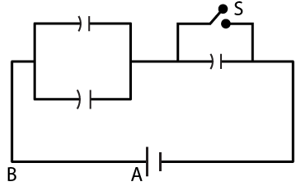Solution:

Case 1: Switch S is open.

The equivalent capacitance = Ceq = 2CxC/3C = (2/3) x 5 μF

And the charge supplied by battery: Q1 = Ceq V

=> Q1 = (2/3) x 5 x 50 = 500/3 μC

case 2: Switch S is Closed.

No current will flow through capacitor at switch. Now there will be only two capacitors which are in parallel. Thus, equivalent capacitance = Ceq = C1 + C2

= 5+5 = 10 μF

And the charge supplied by battery: Q2 = Ceq V = 10 x 50 = 500 μC

Note: Here Q1 will be negative because the capacitor is discharging.

Now,

The charge flows from A to B is:

Qnet = Q1 + Q2 = – 500/3 + 500 = -3.3 x 10-4 C

Question 21: The particle P shown in figure has a mass of 10 mg and a charge of –0.01 μC. Each plate has a surface area 100 cm2 on one side. What potential difference V should be applied to the combination to hold the particle P in equilibrium?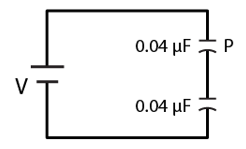Solution:

m = 10 mg

Charge of the particle = q = -0.01 μC = -0.01 x 10-6 C

Area of each capacitor plates = A = 100 cm2 = 100 x 10-4 m2

capacitance of each pair of the parallel capacitor plates = C = 0.04 μF = 0.04×10-6 F

The particle is balanced when electrical force on it balanced by its weight.

=> mg = qE = qV’/d …(1)

Where, V’ = potential difference across the capacitor contained by particle

We know, capacitance of capacitor = C = Єo A/d …(2)

using (2) in (1) , we get

mg = qV’x C/Єo A

or V’ = mg Єo A/qC

= [10-6 x 9.8 x 8.85×10-12x100x10-4]/[0.01×10-6x0.04×10-6]

= 21.68 mV

AS value of both the capacitors are equal, so

V = 2V’ = 2×21.68 = 43 mV (approx)

Question 22: Both the capacitors shown in figure are made of square plates of edge a. The separations between the plates of the capacitors are d1 and d2 as shown in the figure. A potential difference V is applied between the points a and b. An electron is projected between the plates of the upper capacitor along the central line. With what minimum speed should the electron be projected so that it does not collide with any plate? Consider only the electric forces.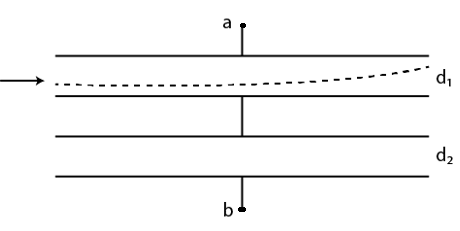Solution:

Let us rearranged the figure,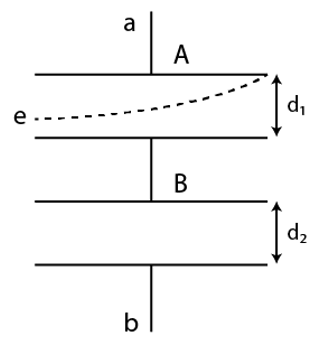Area of each plates = a2, where a = length of each plate

Horizontal distance , x = ut …(1)

Let E be the electric field inside the capacitor

Acceleration of the electron = qE/m

Vertical distance = y = (1/2)qE/m t2 = (1/2)qE/m (x/u) 2 ……

Y = d1/2 and x = a

d1/2 = (1/2) (qE/m) (a/u) 2 …..(2)

capacitance of two capacitors which are connected in series:

C1 = Єoa2/d1 and C2 = Єoa2/d2

Ceq = C1C2/(C1+C2)

On putting values, we get

Ceq = Єoa2/(d1 + d2)

Total charge on the system of capacitors = Q = Ceq V = Єoa2V/(d1 + d2)

The potential difference across the capacitor containing the electron = V = Q/C1

On substituting the values of Q and C1, we get

V = d1/(d1+d2)

Magnitude of electric field inside the capacitor = E = V/d1 = V/(d1+d2)

Charge on electron q is shown as e

On putting the values of q and E in (2)

d1/2 = (1/2) qV/m( d1+d2) x (a/u)2

on rearranging, we have the value of v,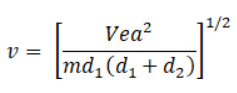Question 23: The plates of a capacitor are 2.00 cm apart. An electron-proton pair is released somewhere in the gap between the plates and it is found that the proton reaches the negative plate at the same time as the electron reaches the positive plate. At what distance from the negative plate was the pair released?

Solution:

Let “E” be the electric field inside the capacitor.

The distance in between the capacitor plates is 2 cm (Given)

Let mp, me be the mass and qp, qe be the charge of proton and electron respectively.

Magnitude of acceleration of the electron= ae = (qeE)/me

Magnitude of acceleration of the proton = ap = (qpE)/mp

Vertical distance travelled by proton to reach the negatively charged plate, say x cm. Thus, (2-x) cm distance travelled by electron.

This implies,

x = (1/2) (qpE)/mp x t2 and 2 – x = (1/2) (qeE)/me x t2

Where “t” be the time taken by the electron and proton to reach the positive and negative plates, respectively.

[Initial velocities of the proton and electron are zero.]

Divide above equations, to find the distance travelled by proton: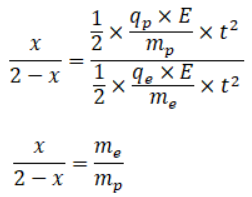[Since charge of proton qp = charge of electron qe]

Mass of electron, me = 9.1×10-31 kg

Mass of proton, mp = 1.67×10-27 kg

Substituting the above values, we get

x = 1.08 x 10-3 cm

Question 24: Convince yourself that parts (a), (b) and (c) of figure are identical. Find the capacitance between the points A and B of the assembly.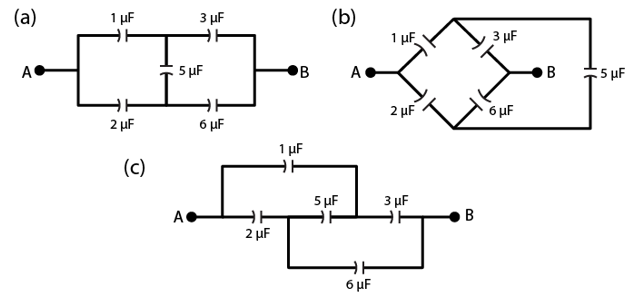Solution:

Given figures (a), (b) and (c) are identical. In this bridge circuit, two electrical paths are branched in parallel between the same potential difference, but are bridged by a third path, from intermediate points.

Here capacitors of 1 µF and 2 µF are connected to terminal A and the 5 µF capacitor and capacitors of 3 µF and 6 µF are connected to terminal B and the 5 µF capacitor.

Form given, No current will flow through the 5 µF capacitor as bridge is in balance

To simplify the circuit, let us remove 5 µF capacitor from the circuit.

Now, remaining 1 µF and 3 µF capacitors are in one series. And 2 µF and 6 µF capacitors are in another series. Results of both the series are in parallel.

The equivalent capacitance in each of the arrangement: Ceq = (1×3)/(1+3) + (2×6)/(2+6) = 2.25 μF

Thus, for all cases, equivalent capacitance of the circuit remains constant.

Question 25: Find the potential difference Va – Vb between the points a and b shown in each part of the figure.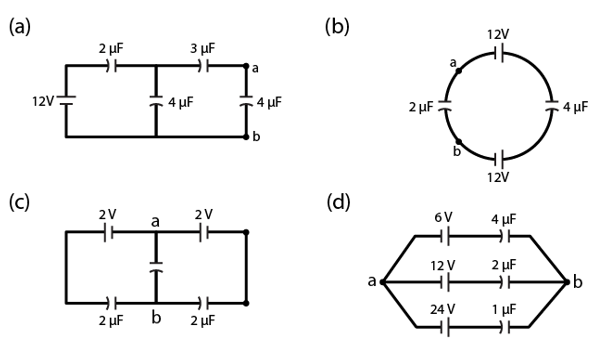Solution:

(a)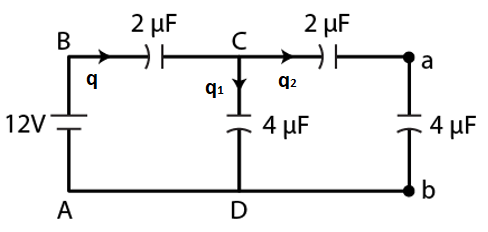q = q1 + q2 …(1)

Let’s apply Kirchhoff’s voltage law in loop CabDC and in loop DCBAD

q2/2 + q2/4 – q1/4 = 0

=> 3q2 = q1 …(2)

And

q/2 + q1/4 – 12 = 0

=> 3q1 + 2q2 = 48 …(3)

Solving (2) and (3), we get

q2 = 48/11

Again,

Va – Vb = q2/4μF = 48/44 = (12/11) V

(b)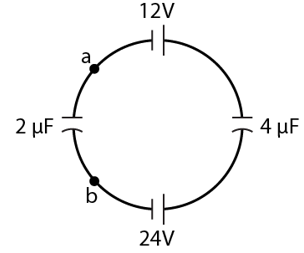q/2 + q/4 – 24 – 12 = 0

Where q be the charge in the loop.

3q/4 = 12

q= 16 μC

Va – Vb = -q/2 = -8V (putting value of q)

(c)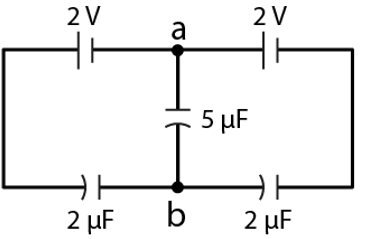Va – Vb = 2 – (2-q)/2μF

In the loop, we have

2+2-q/2-q/2 = 0

=>Q = 4C

Va – Vb = 2 – 4/2 = 0

(d)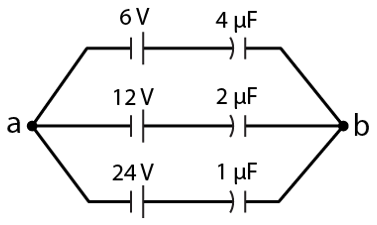Net charge and net capacitance through all the branches:

q = 3×24 = 72 μC and C = 4+2+1 = 7μF

Total potential difference between a and b V = q/C = 72/7 = -10.3 V

[-ve sign due to negative terminals of the batteries connected to point a]